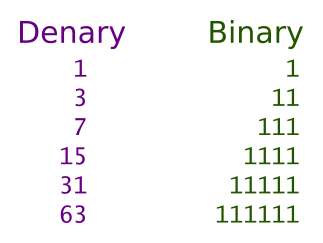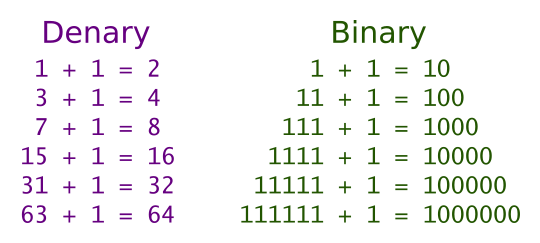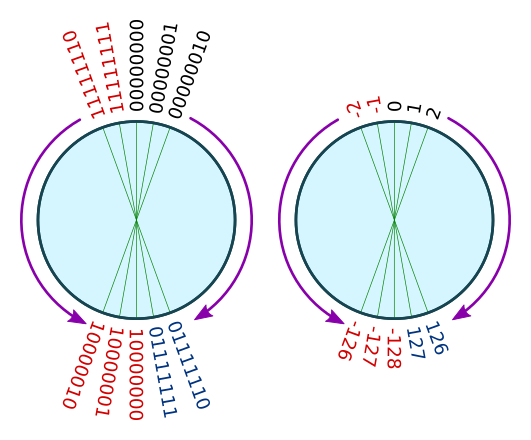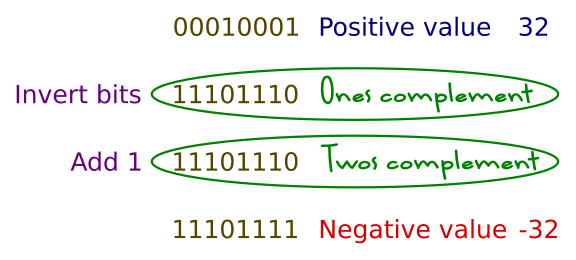# Negative binary numbers

Martin McBride, 2017-02-21
Tags binary addition subtraction negative sign bit ones complement twos complement
Categories data representation numbers

You know how to use binary to represent numbers, but up until now you might only have used positive numbers.

How do we use binary to represent binary numbers?

## Overflow

To understand negative numbers in binary, you need to know about number overflow, and for that we need to look at some patterns in how binary numbers work.

For example let's look at the denary numbers 1, 3, 7, 15... These are all numbers which are one less than an exact power of 2. If we write down the binary values they follow a pattern:Every number which is one less than a power of 2 is made up entirely of ones.

What happens if we add one to each of these numbers is also interesting:So, if we start with a binary number that contains n ones, and add one, we end up with a binary number which contains a one followed by n zeros.

Now consider the number 11111111 binary (255 denary). This is the largest number that can be stored in a single byte. What happens if we add one to it?In this case, we started out with 8 ones, when we add 1 to it, we get a a one followed by 8 zeros. But a byte can only hold 8 bits, so the 9th bit (the 1) drops off the end. We end up with the result (for 8 bit numbers):

255 + 1 = 0


This is called overflow, which means that a number gets too big and wraps around to zero.

Bytes wrap around when the count reaches 255 (2 to the power 8, minus 1). 16-bit words wrap around when the count reaches 65535 (2 to the power 16, minus 1). 32-bit and 64-bit words wrap around at larger values.

Overflow is not an error, it is how things are supposed to work in a computer.

However, overflow can cause errors if you don't take it into account when you are programming. Most languages allow you to choose whether an integer is stored as a byte, or 16 bit word, or 32 bit word or larger. You must choose a size which is large enough to hold the biggest number your program will ever use.

## The number circle

We will only talk about bytes for the rest of this topic, but the ideas can be easily extended to larger word sizes.

If we start with a byte value of 0, and keep counting up, we eventually reach a count of 255. If we count one more we go back to 0 (this is a bit like a clock , it starts at 1, counts up to 12, then resets to 1 again). Here is an illustration, showing the binary values and equivalent denary values:Starting at 0 we count up as follows:

Binary      Denary
00000000    0
00000001    1
00000010    2
...
11111110    254
11111111    255
00000000    0         Starts again at 0


## Negative numbers

Now we understand how overflow works, we can consider this question - what happens if you add 255 to a number?

As an example what is 7 + 255? Well, we know that there are 256 numbers on our number circle. So if we start at 7 and move round 255 places, we will almost complete the whole circle and arrive back were we started. But we will fall one short, arriving at 6 rather than 7. So, when we are dealing with bytes, adding 255 has the same effect as subtracting 1. We could say that binary 11111111 represents -1:

Binary      Denary
11111111    -1


There isn't really anything magical going on here - all we are saying is that going 255 places clockwise has the same effect as going 1 place anticlockwise. We can continue this pattern. Adding 254 is the same as subtracting 2, etc.

This pattern continues as we count down:

Binary      Denary
11111111    -1
11111110    -2
11111101    -3
11111100    -4
...
10000001    -127
10000000    -128


This leaves binary codes 00000000 to 01111111 to represent positive numbers:

Binary      Denary
00000000    0
00000001    1
00000010    2
...
01111110    126
01111111    127


We can now draw a new number cycle where the binary codes going clockwise from 0 represent positive numbers, and the binary codes going anticlockwise from 0 represent negative numbers:## The sign bit

One thing you might have noticed is that all negative numbers have the most significant bit (bit 7) set to 1, and all positive numbers have the most significant bit set to 0. We call this bit the sign bit and it is a quick way to check if a number of negative.

## Signed and unsigned numbers

We have seen 2 different number circles. These represent signed and unsigned numbers:

• Unsigned numbers - byte values 00000000 to 11111111 represent values 0 to 255 denary.
• Signed numbers - byte values 00000000 to 01111111 represent values 0 to 127 denary, byte values 11111111 down to 10000000 represent values -1 to -128.

Many languages give you a choice to use signed or unsigned values. You should use signed values for normal calculations, but you can use unsigned numbers if you definitely know that the values won't be negative.

You must not mix signed and unsigned values within the same calculation. Stick to one or the other.

## Calculating negative values

How would you work out the byte value of, for example, -33 denary?

Well it is quite easy. We need to start with the binary value of +33, which is 00010001.

1. We find the the bitwise inverse of the value. To do this, simply invert each bit (change 0 to 1, or 1 to 0). This gives 11101110. This is called the ones complement.
2. We add 1 to the ones complement. This gives 11101111. This is called the twos complement.

The twos complement is the negative of the original value, so -33 denary equals 11101111.We can verify this because, using byte arithmetic:

00010001 + 11101111 = 00000000


Why does this work? Well, look what happens if you add a number to its ones complement:

00010001 + 11101110 = 11111111


This will always be the case, because wherever there is a 1 in the original value, there will be a 0 in the ones complement, and vice versa. So the sum will always be 11111111. If we add 1 to this we get 0:

00010001 + (11101110 + 1) = 11111111 + 1 = 0